# RD Sharma Solutions - Chapter 25 - Data Handling-III (Part - 1), Class 8, Maths Notes | Study RD Sharma Solutions for Class 8 Mathematics - Class 8

## Class 8: RD Sharma Solutions - Chapter 25 - Data Handling-III (Part - 1), Class 8, Maths Notes | Study RD Sharma Solutions for Class 8 Mathematics - Class 8

The document RD Sharma Solutions - Chapter 25 - Data Handling-III (Part - 1), Class 8, Maths Notes | Study RD Sharma Solutions for Class 8 Mathematics - Class 8 is a part of the Class 8 Course RD Sharma Solutions for Class 8 Mathematics.
All you need of Class 8 at this link: Class 8

PAGE NO 25.12:

Question 1:

The number of hours, spent by a school boy on different activities in a working day, is given below:

 Activities Sleep School Home Play Others Total Number of hours 8 7 4 2 3 24

Present the information in the form of a pie-chart.

Ans.

We know:
Central angle of a component = (component value / sum of component values × 360)
Here, total number of hours = 24
Thus, the central angle for each component can be calculated as follows:

 Activity Number of hours Sector angle Sleep 8 8/24 × 360 = 120o School 7 7/24 × 360 = 105o Home 4 4/24 × 360 = 60o Play 2 2/24 × 360 = 30o Others 3 3/24 × 360 = 45o

Now, the pie chat that represents the given data can be constructed by following the steps given below:
Step 1 : Draw a circle of an appropriate radius.
Step 2 : Draw a vertical radius of the circle drawn in step 1.
Step 3 : Choose the largest central angle. Here, the largest central angle is 120o. Draw a sector with the central angle 120o in such a way that one of its radii coincides with the radius drawn in step 2 and another radius is in its counter-clockwise direction.
Step 4 : Construct other sectors representing other items in the clockwise direction in descending order of magnitudes of their central angles.
Step 5 : Shade the sectors with different colours and label them as shown as in the figure below.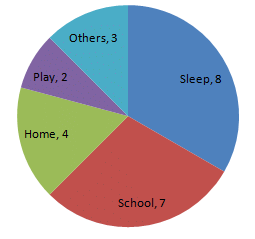PAGE NO 25.12:

Question 2:

Employees of a company have been categorized according to their religions as given below:

 Religions Hindu Muslim Sikh Christian Total Number of workers 420 300 225 105 1080

Draw a pie-chart to represent the above information.

Ans.

We know:
Central angle of a component = (component value / sum of component values × 360ο)
Here, total number of workers = 1050
Thus, the central angle for each component can be calculated as follows:

 Religion Number of workers Sector angle Hindu 420 420/1050 × 360 = 144 Muslim 300 300/1050 × 360 = 102.9 Sikh 225 225/1050 × 360 = 77.14 Christian 105 105/1050 × 360 = 36

Note: The total number of workers is 1050, not 1080.

Now, the pie chat that represents the given data can be constructed by following the steps below:
Step 1 : Draw circle of an appropriate radius.
Step 2 : Draw a vertical radius of the circle drawn in step 1.
Step 3 : Choose the largest central angle. Here, the largest central angle is 144o. Draw a sector with the central angle 144o in such a way that one of its radii coincides with the radius drawn in step 2 and another radius is in its counter clockwise direction.
Step 4 : Construct other sectors representing other items in the clockwise direction in the descending order of magnitudes of their central angles.
Step 5 : Shade the sectors with different colours and label them as shown as in the figure below.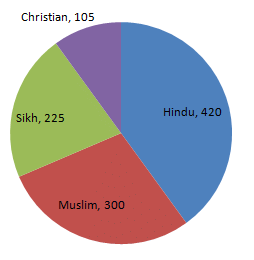PAGE NO 25.12:

Question 3:

In one day the sales (in rupees) of different items of a baker's shop are given below:

 Items Ordinary bread Fruit bread Cakes and Pastries Biscuits Others Total Sales (in Rs) 260 40 100 60 20 480

Draw a pie-chart representing the above sales.

Ans.

We know:
Central angle of a component = (component value/sum of component values × 360)
Here, total sales = Rs 480
Thus, the central angle for each component can be calculated as follows:

 Item Sale (in Rs) Sector angle Ordinary bread 260 260/480 × 360 = 195 Fruit bread 40 40/480 × 360 = 30 Cakes and pastries 100 100/480 × 360 = 75 Biscuits 60 60/480 × 360 = 45 Others 20 20/480 × 360 = 15

Now, the pie chat representing the given data can be constructed by following the steps below:
Step 1 : Draw circle of an appropriate radius.
Step 2 : Draw a vertical radius of the circle drawn in step 1.
Step 3 : Choose the largest central angle. Here, the largest central angle is 195o. Draw a sector with the central angle 195o in such a way that one of its radii coincides with the radius drawn in step 2 and another radius is in its counter clockwise direction.
Step 4 : Construct other sectors representing other items in the clockwise direction in the descending order of magnitudes of their central angles.
Step 5 : Shade the sectors with different colours and label them, as shown as in the figure below.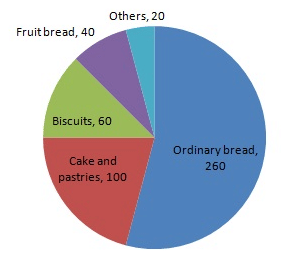PAGE NO 25.12:

Question 4:

The following data shows the expenditure of a person on different items during a month. Represent the data by a pie-chart.

 Items of expenditure Rent Education Food Clothing Others Amount (in Rs) 2700 1800 2400 1500 2400

Ans.

We know:
Central angle of a component = (component value/sum of component values × 360)
Here, total amount = Rs 10800
Thus, the central angle for each component can be calculated as follows:

 Item Amount (in Rs) Sector angle Rent 2700 2700/10800 × 360 = 90 Education 1800 1800/10800 × 360 = 60 Food 2400 2400/10800 × 360 = 80 Clothing 1500 1500/10800 × 360 = 50 Others 2400 2400/10800 × 360 = 80

Total : 10800 (in Rs)

Now, the pie chat representing the given data can be constructed by following the steps below:
Step 1 : Draw circle of an appropriate radius.
Step 2 : Draw a vertical radius of the circle drawn in step 1.
Step 3 : Choose the largest central angle. Here, the largest central angle is 90o. Draw a sector with the central angle 90oin such a way that one radius coincides with the radius drawn in step 2 and another radius is in its counter clockwise direction.
Step 4 : Construct other sectors representing other items in the clockwise direction in descending order of magnitudes of their central angles.
Step 5 : Shade the sectors with different colours and label them, as shown as in the figure below.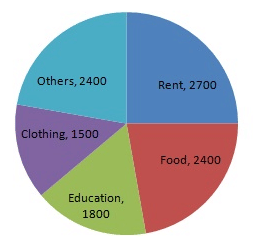PAGE NO 25.12:

Question 5:

The percentages of various categories of workers in a state are given in the following table.

 Categoies Culti-vators Agricultural Labourers Industrial Workers Commercial Workers Others % of workers 40 25 12.5 10 12.5

Present the information in the form a pie-chart.

Ans.

We know:
Central angle of a component = (component value/sum of component values × 360)
Here, total percentage of workers = 100
Thus, the central angle for each component can be calculated as follows:

 Category Percentage of workers Sector angle Cultivators 40 40/100 × 360 = 144 Agricultural labourers 25 25/100 × 360 = 90 Industrial workers 12.5 12.5/100 × 360 = 45 Commercial workers 10 10/100 × 360 = 36 Others 12.5 12.5/100 × 360 = 45

Now, the pie chat representing the given data can be constructed by following the steps below:
Step 1 : Draw circle of an appropriate radius.
Step 2 : Draw a vertical radius of the circle drawn in step 1.
Step 3 : Choose the largest central angle. Here, the largest central angle is 144o. Draw a sector with the central angle 144o in such a way that one of its radii coincides with the radius drawn in step 2 and another radius is in its counter clockwise direction.
Step 4 : Construct other sectors representing other items in the clockwise direction in descending order of magnitudes of their central angles.
Step 5 : Shade the sectors with different colours and label them, as shown as in figure below.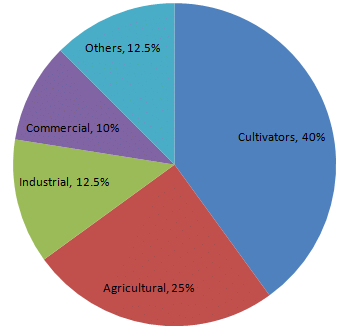PAGE NO 25.12:

Question 6:

The following table shows the expenditure incurred by a publisher in publishing a book:

 Items Paper Printing Binding Advertising Miscellaneous Expenditure (in%) 35% 20% 10% 5% 30%

Present the above data in the form of a pie-chart.

Ans.

We know:
Central angle of a component = (component value/sum of component values × 360)
Here the total % of expenditures = 100%
Thus the central angle for each component can be calculated as follows:

 Item Expenditure (in %) Sector angle Paper 35 35/100 × 360 = 126 Printing 20 20/100 × 360 = 72 Binding 10 10/100 × 360 = 36 Advertising 5 5/100 × 360 = 18 Miscellaneous 30 30/100 × 360 = 108

Now, the pie chat representing the given data can be constructed by following the steps below:
Step 1 : Draw circle of an appropriate radius.
Step 2 : Draw a vertical radius of the circle drawn in step 1.
Step 3 : Choose the largest central angle. Here, the largest central angle is 126o. Draw a sector with the central angle 126o in such a way that one of its radii coincides with the radius drawn in step 2 and another radius is in its counter clockwise direction.
Step 4 : Construct other sectors representing other items in the clockwise direction in descending order of magnitudes of their central angles.
Step 5 : Shade the sectors with different colours and label them, as shown as in figure below.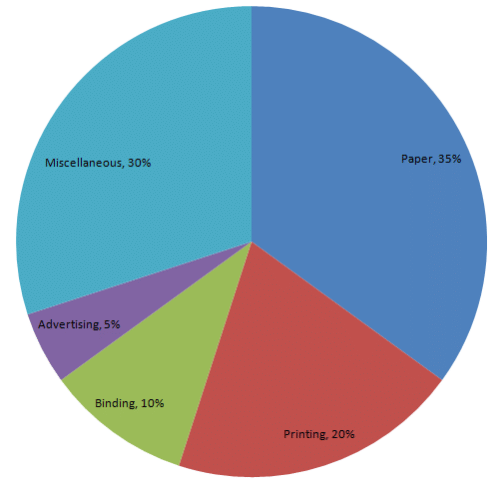PAGE NO 25.12:

Question 7:

Percentage of the different products of a village in a particular district are given below. Draw a pie-chart representing this information.

 Items Wheat Pulses Jwar Grounnuts Vegetables Total % 12531253 12561256 252252 503503 253253 100

Ans.

We know:
Central angle of a component = (component value/sum of component values × 360ο)
Here, the total % of items = 100
Thus, the central angle for each component can be calculated as follows:

 Item In % Sector angle Wheat 125/3 41.66 41.66/100 × 360 = 149.97 Pulses 125/6 20.83 20.83/100 × 360 = 74.98 Jwar 25/2 12.5 12.5/100 × 360 = 45 Groundnuts 50/3 16.66 16.66/100 × 360 = 59.97 Vegetables 25/3 8.33 8.33/100 × 360 = 29.98

Now, the pie chat representing the given data can be constructed by following the steps below:
Step 1 : Draw circle of an appropriate radius.
Step 2 : Draw a vertical radius of the circle drawn in step 1.
Step 3 : Choose the largest central angle. Here the largest central angle is 149.97o. Draw a sector with the central angle 149.97o in such a way that one of its radii coincides with the radius drawn in step 2 and another radius is in its counter clockwise direction.
Step 4 : Construct other sectors representing other items in the clockwise sense in descending order of magnitudes of their central angles.
Step 5 : Shade the sectors with different colours and label them, as shown as in the figure below.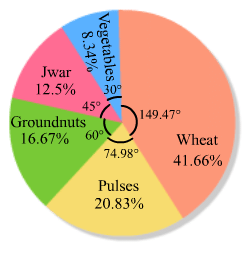PAGE NO 25.13:

Question 8:

Draw a pie-diagram for the following data of expenditure pattern in a family:

 Items Food Clothing Rent Education Unforeseen events Midicine Expenditure (in percent) 40% 20% 10% 10% 15% 5%

Ans.

We know:
Central angle of a component = (component value/sum of component values × 360ο)
Here, the total % of items = 100
Thus, central angle for each component can be calculated as follows:

 Item Expenditure Sector angle Food 40% 40/100 × 360 = 144 Clothing 20% 20/100 × 360 = 72 Rent 10% 10/100 × 360 = 36 Education 10% 10/100 × 360 = 36 Unforeseen events 15% 15/100 × 360 = 54 Medicine 5% 5/100 × 360 = 18

Now, the pie chat representing the given data can be constructed by following the steps below:
Step 1 : Draw circle of an appropriate radius.
Step 2 : Draw a vertical radius of the circle drawn in step 1.
Step 3 : Choose the largest central angle. Here the largest central angle is 144o. Draw a sector with the central angle 144o in such a way that one of its radii coincides with the radius drawn in step 2 and another radius is in its counter clockwise direction.
Step 4 : Construct other sectors representing other items in the clockwise sense in descending order of magnitudes of their central angles.
Step 5 : Shade the sectors with different colours and label them, as shown as in figure below.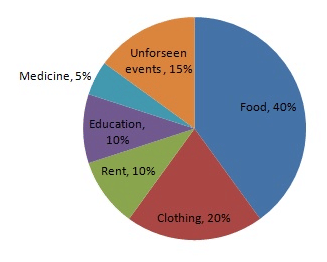PAGE NO 25.13:

Question 9:

Draw a pie-diagram of the areas of continents of the world given in the following table:

 Continents Asia U.S.S.R Africa Europe Noth America South America Australia Area  (in million sq. km) 26.9 20.5 30.3 4.9 24.3 17.9 8.5

Ans.

We know:
Central angle of a component = (component value/sum of component values × 360)
Here, total area in million sq km = 133.3
Thus, the central angle for each component can be calculated as follows:

 Continent Area (in million sq. km) Sector angle Asia 26.9 26.9/133.3 × 360 = 72.6 U.S.S.R 20.5 20.5/133.3 × 360 = 55.4 Africa 30.3 30.3/133.3 × 360 = 81.8 Europe 4.9 4.9/133.3 × 360 = 13.2 North America 24.3 24.3/133.3 × 360 = 65.6 South America 17.9 17.9/133.3 × 360 = 48.3 Australia 8.5 8.5/133.3 × 360 = 23

Now, the pie chat representing the given data can be constructed by following the steps below:
Step 1 : Draw circle of an appropriate radius.
Step 2 : Draw a vertical radius of the circle drawn in step 1.
Step 3 : Choose the largest central angle. Here the largest central angle is 81.8o. Draw a sector with the central angle 81.8o in such a way that one of its radii coincides with the radius drawn in step 2 and another radius is in its counter clockwise direction.
Step 4 : Construct other sectors representing other items in the clockwise sense in descending order of magnitudes of their central angles.
Step 5 : Shade the sectors with different colours and label them, as shown as in figure below.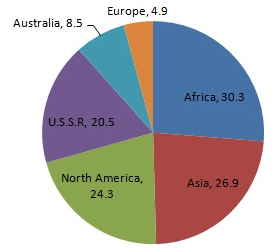PAGE NO 25.13:

Question 10:

The following data gives the amount spent on the construction of a house. Draw a pie diagram.

 Items Cement Timber Bricks Labour Steel Miscellaneous Expenditure  (in thousand Rs) 60 30 45 75 45 45

Ans.

We know:
Central angle of a component = (component value/sum of component values × 360)
Here. the total expenditures = 300 (in thousand Rs)
Thus the central angle for each component can be calculated as follows:

 Item Expenditure(in thousand Rs) Sector angle Cement 60 60/300 × 360 = 72 Timber 30 30/300 × 360 = 36 Bricks 45 45/300 × 360 = 54 Labour 75 75/300 × 360 = 90 Steel 45 45/300 × 360 = 54 Miscellaneous 45 45/300 × 360 = 54

Total expenditure: 300 (in thousand Rs)

Now, the pie chat representing the given data can be constructed by following the steps below:
Step 1 : Draw circle of an appropriate radius.
Step 2 : Draw a vertical radius of the circle drawn in step 1.
Step 3 : Choose the largest central angle. Here the largest central angle is 90o. Draw a sector with the central angle 90oin such a way that one of its radii coincides with the radius drawn in step 2 and another radius is in its counter clockwise direction.
Step 4 : Construct other sectors representing the other items in the clockwise direction in descending order of magnitudes of their central angles.
Step 5 : Shade the sectors with different colours and label them, as shown as in figure below.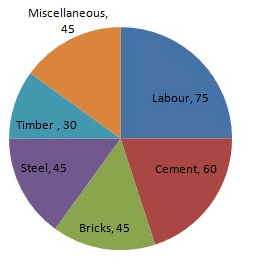PAGE NO 25.13:

Question 11:

The following table shows how a student spends his pocket money during the course of a month. Represent it by a pie-diagram.

 Items Food Entertainment Other expenditure Savings Expenditure 40% 25% 20% 15%

Ans.

We know:
Central angle of a component = (component value/sum of component values × 360)
Here, total expenditure = 100%
Thus, central angle for each component can be calculated as follows:

 Item Expenditure  (in %) Sector angles Food 40 40/100 × 360 = 144 Entertainment 25 25/100 × 360 = 90 Other expenditures 20 20/100 × 360 = 72 Savings 15 15/100 × 360 = 54

Now, the pie chat representing the given data can be constructed by following the steps below:
Step 1 : Draw circle of an appropriate radius.
Step 2 : Draw a vertical radius of the circle drawn in step 1.
Step 3 : Choose the largest central angle. Here the largest central angle is 144o. Draw a sector with the central angle 144o in such a way that one of its radii coincides with the radius drawn in step 2 and another radius is in its counter clockwise direction.
Step 4 : Construct other sectors representing the other items in the clockwise sense in descending order of magnitudes of their central angles.
Step 5 : Shade the sectors with different colours and label them, as shown as in figure below.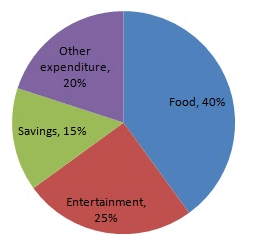The document RD Sharma Solutions - Chapter 25 - Data Handling-III (Part - 1), Class 8, Maths Notes | Study RD Sharma Solutions for Class 8 Mathematics - Class 8 is a part of the Class 8 Course RD Sharma Solutions for Class 8 Mathematics.
All you need of Class 8 at this link: Class 8Use Code STAYHOME200 and get INR 200 additional OFF

## RD Sharma Solutions for Class 8 Mathematics

88 docs

Track your progress, build streaks, highlight & save important lessons and more!

,

,

,

,

,

,

,

,

,

,

,

,

,

,

,

,

,

,

,

,

,

,

,

,

,

,

,

;